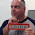## Monday, March 14, 2022

### SQL has a constant for piToday is 3/14 in *MDY format, it is called pi day in recognition of the mathematical constant of the same name. Therefore, I thought that this would be the ideal occasion to write about the SQL scalar function PI().

Many years ago when I was at school, I was taught that pi is the fraction 22/7. This is not exactly true. The ancient Greek mathematician Archimedes stated that pi, or π in Greek, is a number be approximated as being between the fractions 223/71 and 22/7. It is not exactly 22/7, but that is considered the most popular approximation.

SQL does better than any approximation. If I use the scalar function PI() I am given the true value of pi to 15 decimal places.

I say PI() as the function is not passed any parameters. Then why should it as pi is a constant.

How does PI() compare to the approximations? As I am using ACS's Run SQL Scripts I would just use the following statement:

 ```VALUES PI(),22/7.0,223/71.0 ```

This returns the following results:

 ```00001 ----------------- 3.141592653589793 3.142857142857143 3.140845070422535 ```

As you can see the approximations are close, but not pi.

I am sure you noticed that in my statement the approximation calculation divisor's have a decimal place. Why do I do this? It due to the way SQL handles numbers. If I do not give a number of decimal place, then the numbers are considered integers.

 ```VALUES 22/7,223/71 ```

When the calculation is performed the results are also integers.

 ```00001 ----- 3 3 ```

Neither result is close to being approximations of pi.

Harking back to my school days I know I used pi calculating the circumference of circles. The formula or doing this calculation is simple:

 ```circumference = π * diameter ```

I have a circle that has a diameter of 10. You can decide if the units are centimeters, inches, feet, meters, yards, etc. Using the above formula I can just:

 ```VALUES PI() * 10 ```

Which returns to me:

 ```00001 ----------------- 31.41592653589793 ```

I don't need that number of decimals places in my result. I only want two, therefore, I can use the ROUND function to return to me only two decimal places and "half adjust" the result.

 ```VALUES ROUND(PI() * 10,2) ```

And I am returned:

 ```00001 ----- 31.42 ```

I think that is a more manageable result.

I can even use PI() in a RPG program to calculate the circumference of a circle:

 ```01 **free 02 dcl-s Diameter packed(5:3) inz(10) ; 03 dcl-s Circumference packed(6:2) ; 04 exec sql SET :Circumference = ROUND(:Diameter * PI(),2) ; 05 dsply ('Circumference = ' + %editc(Circumference:'J')) ; 06 *inlr = *on ; ```

Line 1: If you are not coding in totally free RPG you are just making too much work for yourself.

Lines 2 and 3: These are the variables I will be using. The variable Diameter is initialized with the value 10.

Line 4: I am using a SET SQL statement to receive the result of the calculation into the variable Circumference.

Line 5: I am using the display operation code, DSPLY, to show the value in the variable Circumference. As that variable is number I am using the %EDITC built in function to convert and edit it into a character string.

The following is displayed:

 ```DSPLY Circumference = 31.42 ```

All that is left for me to do is to wish you all a happy pi day!

This article was written for IBM i 7.4, and should work for some earlier releases too.

1.Is it really Pi day though? 3.14, but only if you format your dates mm.dd. If, like UK, you right it dd/mm, the Pi day could fall on 22 July? Or do we just admit there is no consensus, and for the benefit of international relations agree to have two Pi days?

1.I see no reason why there should not be two pi days.

2.Excellent article! I remember being initiated into a fraternity in college where one of the questions was "What is your favorite kind of pie?" The answer of course was Delta Sigma Pi the name of the fraternity. I got it right!

To prevent "comment spam" all comments are moderated.
```